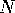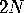GlossaryNext: Background for Further Up: Interactive Methods for Visualizable Previous: References

# Glossary

1. Manifold- A generalization of-dimensional space in which a neighborhood of each point, called its chart, looks like Euclidean space. The charts are related to each other by Cartesian coordinate transformations and comprise an atlas for the manifold. The atlas may be non-trivially connected; there are round-trip tours of a manifold that cannot be contracted to a point. The surface of a donut, called a torus, is a familiar non-trivial 2D manifold.

2. Submanifold, ambient space. A submanifold is a subset of a manifold, its ambient space, for which each point has a chart in which the submanifold looks like a linear subspace of lower dimension. A common knot is a 1-dimensional submanifold of its 3-dimensional ambient space.

3. Homotopy. A continuous deformation of a mathematical object which preserves its topological integrity but may develop self-intersections and even worse singularities. There is a homotopy that takes a teapot to a torus (a sphere with a hole). There is another deforming it to a point.

4. Isotopy. A homotopy of an object produced by a deformation of the ambient space, so therefore the object cannot develop new self-intersections. The deformation of the teapot to a torus is an isotopy, but the deformation to a point is not.

5. Embedding. The parametrization of a submanifold by means of a standard model. A knotted sphere in 4-space is an embedding of the familiar round sphere. Whitney's theorem says that an-dimensional manifold is guaranteed to have an embedding in Euclidean-space.

6. Immersion. A locally (but not globally) smoothly invertible mapping of one manifold into another. The image may have self-intersections; the figure-8 is an immersion of the circle in 2D.

7. Minimal Surface. A surface that locally has the smallest area given a particular topological shape for it, and possibly, constrained by a fixed boundary (soap-films) or prescribed behavior at infinity.

8. Steepest Descent Method. A particular way of guiding an isotopy of an embedded surface to one which minimizes a function that measures its shape. Moving down the gradient of the area function often terminates at a minimal surface.Next: Background for Further Up: Interactive Methods for Visualizable Previous: References

Tamara Munzner
Thu Sep 21 19:17:33 CDT 1995